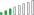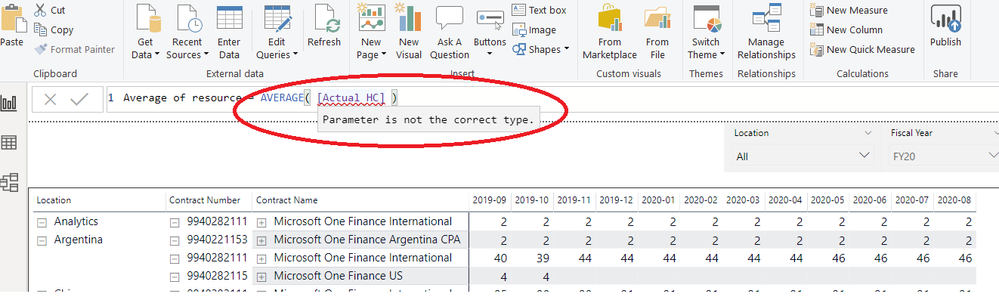cancel
Showing results for
Did you mean:Post Prodigy

## Average of a Measure

Hi,

I need to callculate an average of a Measure.  It the following screen should be  46/47  but not 52

I tried with a formula that you see there but didn't work.

My measure is the one called "Actual HC"

Actual HC = CALCULATE(DISTINCTCOUNT('Resource Actual'[Name]),FILTER('Resource Actual','Resource Actual'[Category]="Hours" && 'Resource Actual'[Quantity]>8))Any ideas ?

Thanks

1 ACCEPTED SOLUTIONResolver III

Ooops, sorry, I forgot that we were working with a measure already. You will need to use AVERAGEX with the <table> parameter identifying your Year-Month column. I think the following will work, but if not, let me know.

AVERAGEX( VALUES( 'YourDateTable'[Year-Month]), [Actual HC] )

where 'YourDateTable' is your date table and [Year-Month] is the column in your date table with the year-month.

https://docs.microsoft.com/en-us/dax/averagex-function-dax

5 REPLIES 5Resolver III

Please share the DAX for [Actual HC].Post Prodigy

Hi @RandyPgh ,

Actual HC = CALCULATE(DISTINCTCOUNT('Resource Actual'[Name]),FILTER('Resource Actual','Resource Actual'[Category]="Hours" && 'Resource Actual'[Quantity]>8))

regardsResolver III

Your illustration in the original post shows the spinners in the top-left of each visualization. This means that they are still loading. Assuming that they look the same after the spinners complete, you would need to change the Average calculation to be

Average of resource = AVERAGE( [Actual HC] )

Then put this "Average of resource" measure into the Field of the card visualization instead of the current "Actual HC". That should change the 52 to a 45.5 (if visualized with decimals).Post Prodigy

Hi @RandyPgh ,

Is not working:Resolver III

Ooops, sorry, I forgot that we were working with a measure already. You will need to use AVERAGEX with the <table> parameter identifying your Year-Month column. I think the following will work, but if not, let me know.

AVERAGEX( VALUES( 'YourDateTable'[Year-Month]), [Actual HC] )

where 'YourDateTable' is your date table and [Year-Month] is the column in your date table with the year-month.

https://docs.microsoft.com/en-us/dax/averagex-function-dax